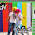### Diminishing Differences Puzzle

Source: Australian Mathematical Society Gazette Puzzle Corner 34

Problem: Begin with n integers x1, . . . , xn around a circle. At each turn, simultaneously replace all of them by the absolute differences

Repeat this process until every number is 0, then stop. Prove that this process always terminates if and only if n is a power of 2.

Shameless plug:
Follow CSE Blog on CSE Blog - Twitter and CSE Blog on Quora. :-)

1.there are two parts to the proof.

part A: the theorem will be proved for case of arithmetic modulo 2. i.e. only 0 and 1, with addition and substraction as usual defined by 0+0=0,1+1=0,1+0=0+1. If we work in normal arithmetic, this is equivalent to saying that we always end up with all even numbers for an arbitrary configuration of initial numbers, iff n is a power of two.

proof of Part A is produced late belowr. But assuming part A is proved, the remaining proof is as under:

proof of only if part : suppose the process always terminates for some n, then for that n the process will obviously always terminate for arithmetic modulo 2 . So, from part A, we conclude that n is a power of 2.

proof of if part: suppose n is power of 2. then from part A, we conclude that by repeating process, we reach a configuration of all even numbers in the circle . Now divide all numbers by an appropriate power of 2 so that we have some odd numbers in the cycle. again from part A, we conclude that by repeated process, we reach a configuration of all even numbers in the circle . again factor out the highest power of two and continue the above procedure. Its clear that the above procedure cannot continue indefinitely, because the initial numbers are finite and at each step, we are dividing by a power of 2. therefore conclusion is ultimately we end with all zero in the circle. now insert back the powers of 2 into the circle wherever required to get a all 0 circle.

Below is produced proof of part A: all arithmetic is modulo 2 . so we are dealing with 0 and 1 only.

proof of only if part.

suppose for some n the process always terminates. Required to prove that n is a power of 2. It suffices if we show that all non unity divisors of n are even.
Suppose n= d*e. where d>1 . consider a configuration of the nos. in circle as under.

[ 1,0,....,0 ,total d numbers] ,[1,0,0,....,0,total d numbers],...., the bracketed sequence repeating e times.

please note that this is a symmetrical situation , involving a identical block of [1, 0,.....0, total d numbers] repeated e times in a circle. please convince yourself , by induction, that after any number of steps, the result will be of same form that is b1,b2,....,bd repeated e times on the circle.

in the last step we get all 0s. so in second last step, we must get all 1s. suppose the numbers in the third last step are
[b1,b2,.....bd] repeated e times on circle. then we must have,

b1=b2+1,b2=b3+1,....bd=b1+1. so b1= b1+ (1+1+1+...d times). 1+1+1+... d times=0, so we conclude that d is even.

so n must be power of 2. this completes proof of only if part.

proof of if part:
suppose n is a power of 2, i.e. n = 2^r. we will prove that after n steps, we get all 0s.

consider a special case where numbers in circle are [1,0,0,....,0] . Its easily seen that it suffices if we prove that for this special case we get a zero after n steps. Just prove by induction that after 2^k steps, where k<r, the result will be that the original 1 will be at its place and in addition there will be another 1 in the circle at a distance of 2^k counterclockwise, other numbers being zeroes. So after 2^(r-1) steps there will be two 1s on the circle with a distance of 2^(r-1) i.e. the second 1 is diagonally situated. After another 2^(r-1) steps , these two 1s will contribute 1 in their respective diagonals, and the result will be all 0s.
this completes proof of if part.

2.http://www.austms.org.au/Publ/Gazette/2014/Mar14/Puzzle.pdf

3.hey, what if the sequence is something like 0,16,0,16,0,16; in that case n is 6 and you get all zeroes.

1.The statement implies that if n is not a power of 2, then you can find at least one sequence {x1,x2,x3 ... xn) for which the process of taking absolute differences does not terminate.